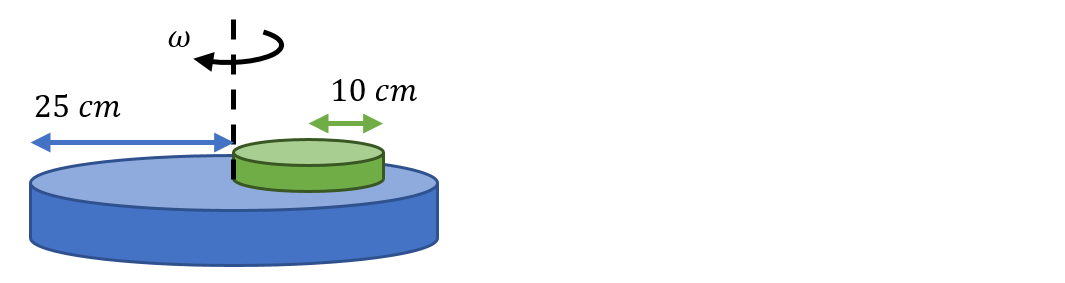# Problem: A smaller disk of mass 0.15 kg sits atop a larger disk of mass 0.5 kg as shown in the figure. If the system rotates about the axis shown with an angular speed of 150 rad/s, what is the moment of inertia of the system?

80% (40 ratings)
###### Problem Details

A smaller disk of mass 0.15 kg sits atop a larger disk of mass 0.5 kg as shown in the figure. If the system rotates about the axis shown with an angular speed of 150 rad/s, what is the moment of inertia of the system?